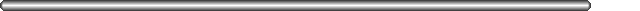# CLASS 8## If-Then.

If-then is pretty simple, just complement the condition and jump OVER the body of the "if" if it's not true.

To translate:

```
/*  six.c  */

d = a;
if ((a + b) > c) {
a += b;
c++;
}
a = c + d;
```
We would get (before filling delay slots):
```
/*  six.s  */

mov     %a_r, %d_r
cmp     %o0, %c_r
ble     next
nop
next:
```
Fill the delay slot with the first prior instruction that doesn't affect the condition code:
```
/*  six1.s  */

cmp     %o0, %c_r
ble     next
mov     %a_r, %d_r
next:
```
This is best. However, sometimes you just can't find a good instruction to fill the delay slot. In that case, using the annulled version, you can fill the delay slot "half the time":
```
/*  six2.s  */

mov     %a_r, %d_r
cmp     %o0, %c_r
ble,a   next
next:
```
Trace the number of instructions executed if the branch is taken, and if the branch isn't taken.

## If-Then-Else.

```
/*  seven.c  */

if ((a + b) >= c)
{
a += b;
c++;
}
else
{
a -= b;
c--;
}
c += 10;
```
Now, as before, we complement the condition and jump over the first block. Don't interchange blocks -- it makes the assembly code too hard to compare to the C code.
```
/*  seven.s  */

cmp     %o0, %c_r
bl      else
nop
ba      next
nop
else:   sub     %a_r, %b_r, %a_r
sub     %c_r, 1, %c_r
```
There are a number of ways to get rid of the nops here. The first nop can be removed using an annulled branch:
```
/*  seven1.s  */

cmp     %o0, %c_r
bl,a    else
sub     %a_r, %b_r, %a_r
ba      next
nop
sub     %a_r, %b_r, %a_r
else:   sub     %c_r, 1, %c_r
```
The second nop can be removed by moving code (eg, the c++ line):
```
/*  seven2.s  */

cmp     %o0, %c_r
bl,a    else
sub     %a_r, %b_r, %a_r
ba      next
sub     %a_r, %b_r, %a_r
else:   sub     %c_r, 1, %c_r
```

### Nesting of loops

Consider the following segment of C code:
```
/*  eight.c  */

for (i =15, i > 3; i--)
{
if (i == 12)
j = 10;
else
j = 12;
}
```

The assembly code for the following is given below :

```
/*  eight.s  */

mov 15, %i_r
loop:
cmp %i_r, 3
ble   exit
nop
cmp %i_r, 12
bne  else
nop
mov 10, %j_r
sub   %i_r, 1, %i_r
ba     loop
nop
else:
mov 12, %j_r
sub   %i_r, 1, %i_r
ba     loop
nop
exit:
```
I leave it as an exercise for you to optimize this piece of code to get rid of all the nops (if possible!!)

## Implementation of the pipeline in SPARC

In the SPARC chip, designers used special logic to make the pipeline appear to be only two-deep.

There are two program counters. %pc and %npc. %npc is always copied to %pc; but %npc is sometimes incremented by four, other times modified. On each cycle, this occurs. One can understand how delay slots work by tracking the contents of pc and npc. Because there are TWO program counters, the effective depth of the SPARC pipeline is 2.

The net effect is that instructions after branches and calls are always executed. They are called "delay slot" instructions.

Clearly, one can always put nops in there. How can this be more useful? Well, it turns out that there is almost always an instruction that can be put there. The instruction cannot be allowed to modify data that affects the branch however!

To fill a delay slot, find an instruction that can be placed immediately before the branch, but doesn't affect the condition tested by the branch.

## Delay Slots in SPARC Assembly

Now we return to the Assembly Language level. We have seen that the result of pipelining in the processor is that the programmer has to be aware of, and plan for, delay slots. Here is how that is done.

Consider translating the following code into assembly:

```
/*  nine.c  */

b = 0;
if (a <= 17)
{
a++;
}
```
Assume we keep b in %l0 and we keep a in %l1. The simplest translation would be:
```
/*  nine.s  */

clr     %l0
subcc   %l1, 17, %g0
bg      end
nop
end:    ! (rest of program here)
```
This code is correct because we have put a "nop" in the delay slot. A nop instruction simply does nothing. However, it is also a waste of the processor's time. The code is less efficient when it contains a nop -- it takes longer to get the job done. What can we do?

We observe that the instruction "clr %l0" doesn't affect the branch condition. So we move it into the delay slot:

```
/*  nine1.s  */

subcc   %l1, 17, %g0
bg      end
clr     %l0
end:    (rest of program here)
```
This code is more efficient, because it only requires 4 clock cycles to execute instead of 5 as before. However, when reading the program, we have to read it OUT OF ORDER -- we think of the "clr" instruction as happening BEFORE the "bg" instruction.

How should we fill a delay slot in general? We cannot move an instruction into the delay slot that affects the condition codes we wish to test. Find an instruction prior to the delay slot that doesn't affect the branch condition.

One thing you should never do is put a control transfer instruction into a delay slot. Chaos would ensue, as can be seen from tracking the contents of %pc and %npc for such a scenario.

Delay Slots - things to remember:

• When filling delay slots, don't change the results the program computes.
• Fill all possible delay slots; the program is faster with full delay slots.
• When reading the program, you should read the instructions out of order.